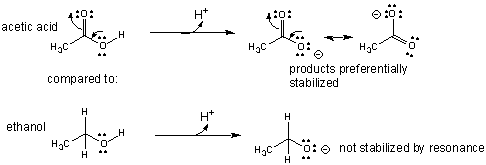What is the priority order of factors like resonance, hybridization, solvation, etc. in determining the relative acidity or basicity of compounds in a list?

Nov 26, 2016

WARNING! Long answer! Here are my thoughts.

Explanation:

Anything that decreases the $\text{X-H}$ bond strength in the acid or stabilizes the conjugate base will increase the acidity and decrease the basicity of the conjugate base.

I would identify the factors in the order 1. $\boldsymbol{\text{R}}$esonance; 2. $\boldsymbol{\text{A}}$tom; 3. $\boldsymbol{\text{D}}$ipole $\boldsymbol{\text{I}}$nductive effect; 4. $\boldsymbol{\text{O}}$rbitals ($\boldsymbol{\text{RADIO}}$).

Strong acids

There are six common strong acids. In order of increasing acidity, they are:

${\text{HNO"_3 < "H"_2"SO"_4 < "HCl" < "HBr" < "HI" < "HClO}}_{4}$

Any strong acid is stronger than most organic acids.

Some factors to consider are:

1. Resonance

I put resonance stabilization of the anion first in the list.

The more resonance contributors a conjugate base has, the stronger the acid will be.

That partly explains why sulfuric acid is stronger than nitric acid.

It also explains why acetic acid ("p"K_"a" ≈5) is a stronger acid than
ethanol ("p"K_"a" ≈ 16).2. Atom

(a) Acidity of H-X increases from left to right on the Periodic Table.

color(white)(mmml)"CH"_4 < color(green)("N")"H"_3 < color(red)("H")_2"O" < "H"color(lime)("F")

"p"K_"a"color(white)(m)~50color(white)(mmll)38color(white)(mml)15.7color(white)(mm)3.2

As the electronegativity of $\text{X}$ increases, the bonding electrons are puled closer to $\text{X}$ and the acidity increases.

The exception is $\text{HF}$, for which the conjugate base $\text{F"^"-}$ is stabilized by solvation by water molecules.

(b) Acidity increases from top to bottom of the Periodic Table.

$\textcolor{w h i t e}{m m m l} \text{HF" < "HCl" < "HBr" < "HI}$
$\text{p"K_"a"color(white)(ml)3.8color(white)(mll)"-8.0"color(white)(mm)"-9.0"color(white)(mll)"-10}$

As you go down a group, the bond length becomes longer and weaker, and the charge density on the ion decreases.

3. $\boldsymbol{\text{D}}$ipoles and $\boldsymbol{\text{I}}$nductive Effects

Electronegative atoms draw negative charge toward themselves.

This weakens the H-X bond and stabilizes the conjugate base.

The effect decreases with distance from the acidic $\text{H}$ atom.(From SlidePlayer)

Alkyl groups are electron-donating groups.

They push electron density onto the $\text{COOH}$ group and destabilize the carboxylate ion.

The more alkyl groups, the weaker the acid.

4. Orbitals

The more $\text{s}$ character in an orbital, the closer the electrons will be to the nucleus.

As we go from ${\text{sp}}^{3}$ (alkane) to ${\text{sp}}^{2}$ (alkene) to $\text{sp}$ (alkyne) hybridization, the stability of the negative charge increases.

Alkynes are remarkably acidic compared to alkanes.Here's a summary of the discussion.